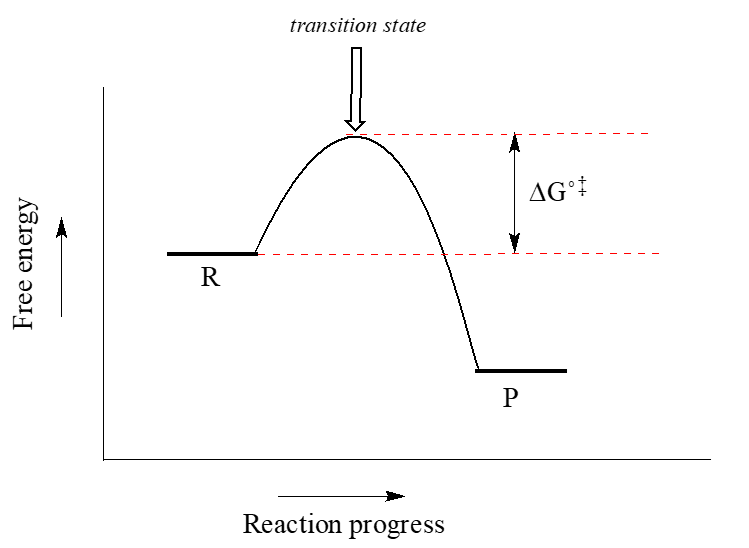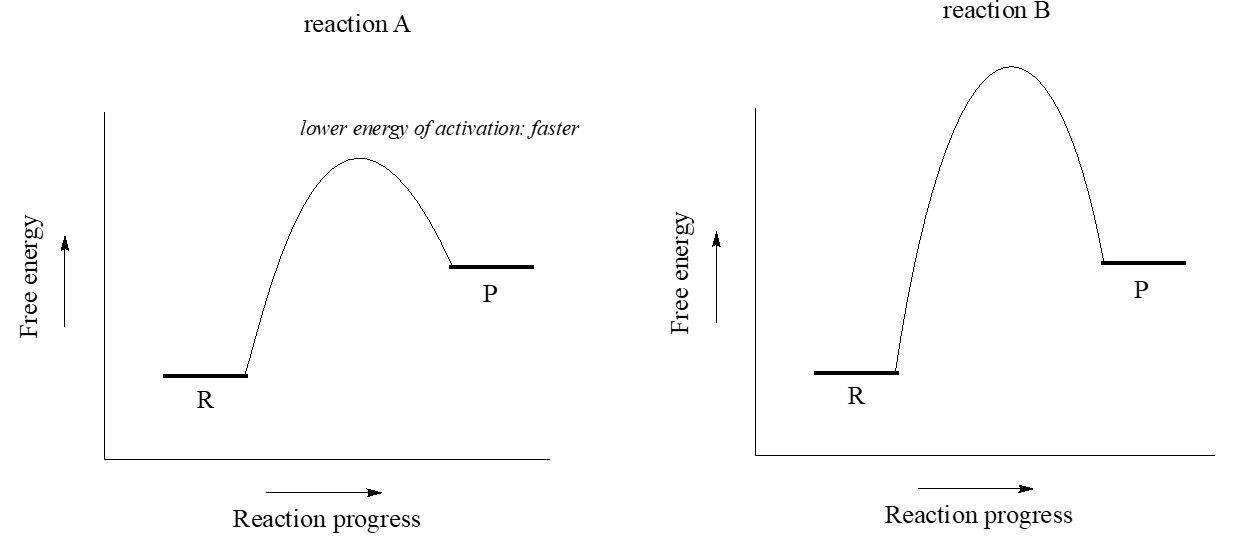# 6.3: A Quick Review of Thermodynamics and Kinetics

$$\newcommand{\vecs}{\overset { \rightharpoonup} {\mathbf{#1}} }$$ $$\newcommand{\vecd}{\overset{-\!-\!\rightharpoonup}{\vphantom{a}\smash {#1}}}$$$$\newcommand{\id}{\mathrm{id}}$$ $$\newcommand{\Span}{\mathrm{span}}$$ $$\newcommand{\kernel}{\mathrm{null}\,}$$ $$\newcommand{\range}{\mathrm{range}\,}$$ $$\newcommand{\RealPart}{\mathrm{Re}}$$ $$\newcommand{\ImaginaryPart}{\mathrm{Im}}$$ $$\newcommand{\Argument}{\mathrm{Arg}}$$ $$\newcommand{\norm}{\| #1 \|}$$ $$\newcommand{\inner}{\langle #1, #2 \rangle}$$ $$\newcommand{\Span}{\mathrm{span}}$$ $$\newcommand{\id}{\mathrm{id}}$$ $$\newcommand{\Span}{\mathrm{span}}$$ $$\newcommand{\kernel}{\mathrm{null}\,}$$ $$\newcommand{\range}{\mathrm{range}\,}$$ $$\newcommand{\RealPart}{\mathrm{Re}}$$ $$\newcommand{\ImaginaryPart}{\mathrm{Im}}$$ $$\newcommand{\Argument}{\mathrm{Arg}}$$ $$\newcommand{\norm}{\| #1 \|}$$ $$\newcommand{\inner}{\langle #1, #2 \rangle}$$ $$\newcommand{\Span}{\mathrm{span}}$$

You may recall from general chemistry that it is often convenient to illustrate the energetics of a chemical reaction with a reaction coordinate diagram. In a reaction coordinate diagram, the vertical axis represents the overall potential energy of the reactants, while the horizontal axis is the ‘reaction coordinate’, tracing from left to right the progress of the reaction from starting reactants R to final products P. (Many students find it helpful to envision the horizontal axis in an reaction coordinate diagram as being analogous to the progress bar at the bottom of a youtube video). The diagram for a typical one-step nucleophilic substitution reaction such as that between hydroxide and methyl chloride might look like this:Despite its apparent simplicity, this diagram conveys some very important ideas about the thermodynamics and kinetics of the reaction. Recall that when we talk about the thermodynamics of a reaction, we are concerned primarily with the difference in energy between reactants (R) and products (P): whether the reaction as a whole is uphill or downhill. When we talk about kinetics, on the other hand, we are concerned with the rate of the reaction: how fast it goes from reactants to products, regardless of whether that transformation is energetically uphill or downhill.

## Thermodynamics

First, a quick review of some key thermodynamics terms. Recall that the standard Gibbs free-energy change of a reaction ($$\Delta G^o$$) is the difference in energy between reactants and products at standard conditions. Gibbs free-energy change is a combination of enthalpy change ($$\Delta H^o$$) and entropy change ($$\Delta S^o$$):

$\Delta G = \Delta H^o - T\Delta S^o$

where

• $$T$$ is the temperature in Kelvin (recall that the Kelvin temperature is simply the Celsius temperature plus 273.15).
• Enthalpy change ($$\Delta H^o$$) is the heat released or absorbed by the reaction.
• Entropy change ($$\Delta S^o$$) is the change in disorder from reactants to products. In a reaction in which one molecule cleaves into two smaller molecules, for example, disorder increases, so $$\Delta S^o$$ is positive.

The equilibrium constant ($$K_{eq}$$) for a reaction is an expression of the relative concentrations of reactants and products after the reaction has reached equilibrium. The equilibrium constant is defined as:Now, let’s review what the above energy diagram tells us about the thermodynamics of the reaction. Note that the energy level of the products is lower than that of the reactants. This tells us that the Gibbs free-energy change for the reaction is negative, and the step is exergonic, or energy releasing. We can also say the reaction is 'thermodynamically favorable', or, more informally, 'downhill'.

Recall from General Chemistry that the standard Gibbs free energy change for a reaction can be related to the reaction's equilibrium constant (Keq) by the equation:

$\Delta G^o = -RT \ln K_{eq}$

where $$R$$ is the gas constant (8.314 J/mol×K) and $$T$$ is the temperature in Kelvin (K).

If you do the math, you see that a negative value for $$\ce{\Delta G_{rnx}^{\circ}}$$ (an exergonic reaction) corresponds to $$\ce{K_{eq}}$$ being greater than 1, an equilibrium constant which favors product formation.

Conversely, an endergonic reaction is one in which the products are higher in energy than the reactants, and energy is absorbed. An endergonic reaction has a positive value of $$\ce{\Delta G _{rnx}^{\circ}}$$, and a $$\ce{K_{eq}}$$ between 0 and 1.Acid-base reactions provide convenient examples of thermodynamically favorable and unfavorable reactions. The reaction of a strong acid like $$\ce{HCl}$$ with a strong base like hydroxide ion, for example, is highly favorable, and has an equilibrium constant much greater than one. The reaction of a weak acid such as acetic acid with a weak base such as water, on the other hand, is unfavorable and has an equilibrium constant that is a very small (much less than 1) positive number: we can visualize this in a reaction coordinate diagram as an 'uphill' reaction, in which $$\ce{\Delta G^{\circ}_{rnx}}$$ is positive.

When talking about exergonic, or 'downhill' reactions, chemists sometimes use the term 'driving force' to describe the chemical factor or factors that drive the reaction from higher energy reactant to lower energy product. Using the 'downhill' analogy again, when water flows downhill, the driving force is gravity. In an exergonic chemical reaction, the driving force generally is based on a combination of two factors: a) the stability of positive and negative charges in the product relative to those in the reactant, and b) the total bond energy in the product relative to the reactant. That may not make a lot of sense right now, but keep it in the back of your mind and we will come back to the idea of driving force when we study different reaction types in greater detail.

Now, let's move to kinetics. Look again at the diagram for an exergonic reaction: although it is ‘downhill’ overall, it isn’t a straight downhill run.First, an ‘energy barrier’ must be overcome to get to the product side. The height of this energy barrier is called the standard free energy of activation ($$\ce{\Delta G^{\circ}_+^+}$$). The activation energy, in combination with the temperature at which the reaction is being run, determines the rate of a reaction: the higher the activation energy, the slower the reaction. At the very top of the energy barrier, the reaction is at its transition state (TS), which you should recall is defined as the highest energy structure in the transition from reactant to product.

Consider the hypothetical reaction reaction coordinate diagrams below.Both reaction A and reaction B are slightly endergonic, or uphill: $$\ce{\Delta G^{\circ}_{rxn}}$$ for both is positive, meaning that $$\ce{K_{eq}}$$ for both is between 0 and 1. However, the energy of activation is higher for reaction B. From this observation, we know that reaction A will proceed faster than reaction B in both forward and reverse directions (temperature and other conditions being equal), so reaction A will reach equilibrium in less time.

##### Exercise 6.3.1

Consider the hypothetical reaction coordinate diagrams below, and assume that they are on the same scale.1. Which of the diagrams describe(s) a reaction with $$\ce{K_{eq} < 1}$$ ?
2. Which of the diagrams describes the fastest reaction?
3. Which of the diagrams describes the reaction with the highest value of $$\ce{K_{eq}}$$?
4. Which of the diagrams describes the reaction with the largest $$\ce{\Delta G _{rnx}^{\circ}_{+}^{+}}$$ for the reverse reaction?
5. Copy the diagram for your answer to part (d), and add a label which graphically illustrates the value of $$\ce{\Delta G _{rnx}^{\circ}_{+}^{+}}$$ for the reaction in the reverse direction.

We turn our attention next to a two-step reaction mechanism, such as the nucleophilic substitution reaction between acetate and tert-butyl chloride. The reaction coordinate diagram for this reaction looks somewhat different from what we have seen until now:Because there are two steps involved, there are also two transition states and two activation energies to consider, as well as a carbocation intermediate (denoted by the letter I). The first, bond-breaking step from R to I, passing over transition state $$TS_1$$, can be depicted as a highly endergonic (uphill) reaction, because the carbocation-chloride ion pair is significantly higher in energy than the reactants. The second step, attack on the carbocation electrophile by the acetate nucleophile and formation of the new carbon-oxygen bond, is a highly exergonic step that passes over a second, lower energy transition state $$TS_2$$. The intermediate (I) is thus depicted as an energy 'valley' (a local energy minimum) situated between the two energy peaks $$TS_1$$ and $$TS_2$$.

Notice that the activation energy for the first step is higher than the activation energy for the second, meaning that the first step is slower. This should make intuitive sense, because the first step involves bond-breaking, separation of charge, and formation of a carbocation, which is high in energy due to lacking a complete octet. Conversely, the second step involves bond-forming and neutralization of charge. In a multi-step reaction, the slowest step - the step with the highest energy of activation - is referred to as the rate-determining step (rds). The rds can be thought of as the 'bottleneck' of the reaction: a factor which affects the rds will affect the overall rate of the reaction. Conversely, a factor which affects a much faster step will not significantly affect the rate of the overall reaction.

##### Exercise 6.3.2

Imagine that you are trying to extinguish a burning campfire using buckets of water filled from a faucet some distance from the fire. It takes 20 seconds to fill a bucket at the faucet, and two seconds to carry the bucket to the fire and dump it on the flames. You have plenty of people to carry the buckets, but only one faucet so you can only fill one bucket at a time.

1. If you double the speed at which you carry the buckets by running instead of walking, what effect will this have on how fast you get water to the fire?
2. If, instead, you realize you can double the speed at which you fill up the buckets by using a second faucet, what effect will this have on how fast you get water to the fire?
3. What is the rate-determining step for the process?
##### Exercise 6.3.3

Use the reaction coordinate diagram below to answer the questions.1. Is the overall reaction endergonic or exergonic in the forward (A to D) direction?
2. How many steps does the reaction mechanism have?
3. How many intermediates does the reaction mechanism have?
4. Redraw the diagram and add a label showing the activation energy for the rate-determining step of the forward reaction.
5. Add a label showing $$\ce{\Delta G^{\circ}_{rxn}}$$ for the reverse reaction (D to A).
6. What is the fastest reaction step, considering both the forward and reverse directions?